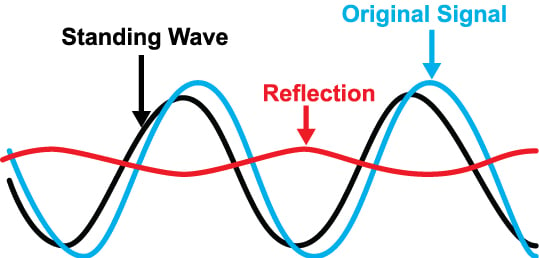# VSWR / Return Loss Calculator

## A calculator designed to compute the VSWR, reflection coefficient, return loss and matching loss in a transmission line

(dB)
(dB)

### OverviewVSWR which stands for Voltage Standing-Wave Ratio is a measure used to determe the severity of standing waves in a transmission line. Standing waves form when there is a mismatch between the line and the load, and is generally undesirable. The reflection coefficient is another measure which is closely related to VSWR. Engineers use the reflection coeffcient to determine how much of the signal propagating on a line is reflected back to the source. Return loss is the measure of how much of the signal is lost when it is reflected back to the source, while matching loss is the loss incurred when there is a great mismatch between the line and the load.

This calculator computes the VSWR, reflection coefficient, return loss and matching loss in a transmission line. Fill in the input field and select the input type and click the "calculate" button to show the values.

### Equations

$$VSWR = \frac{1 + \Gamma}{1 - \Gamma}$$

$$\Gamma = \frac{VSWR-1}{VSWR+1}$$

$$L_{R} = -20log_{10}\Gamma$$

$$L_{M} = -10log_{10}(1-\Gamma^{2})$$

Where:

$$VSWR$$ = voltage standing wave ratio

$$\Gamma$$ = reflection coefficient

$$L_{R}$$ = return loss

$$L_{M}$$ = matching loss

### Applications

VSWR is a parameter used by engineers to know the degree of mismatch between a transmission line and a device (like an antenna or a transmitter/receiver circuit). The presence of standing waves in a transmission line means part of the incident signal is being reflected back to the source. The ratio of the reflected voltage to the incident voltage is the reflection coefficient. The worst case value for the reflection coefficient is one (1). This means that all the incident voltage is reflected back. A reflection coefficient equal to one would result in a VSWR equal to infinity (based on the formula shown above). Therefore, the higher the VSWR, the greater the reflection and consequently the higher the degree of mismatch between the line and the load.

The return loss and mismatch loss are parameters that indicate how much of the signal is lost because of the line mismatch. Engineers are used to seeing power losses in dB, thus having parameters presented in dB is more convenient than ratios (like what the VSWR and reflection coefficient are). For example, instead of saying that the reflection coefficient in the line is 0.8, an engineer would rather say that the signal suffered a return loss of 1.938 dB.

Textbook - Standing Waves and Resonance

Textbook - Impedance Transformation

Textbook - Maximum Power Transfer• C
chucurucu January 16, 2018

These calculator pages and great and a very useful resource. Thank you for providing them and all of the other content on the site. I would like to point out however, that the denominator on the RH side of the equation given for VSWR should be (1 - Γ) instead of (Γ -1). Again, great site and keep up the good work!

Like.
•RK37 January 16, 2018
Thank you for pointing this out! I corrected the equation.
Like.
•Picwiz October 17, 2021

Why the calculator doesn’t work?

Like.